## Solution to 1986 Problem 77

 The motion of the particle is described by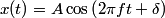\begin{align*}x(t) = A \cos \left(2 \pi f t + \delta \right)\end{align*}So, the particle's velocity is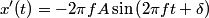\begin{align*}x'(t) = -2 \pi f A \sin \left(2 \pi f t + \delta \right)\end{align*}When x(t) = A/2 ,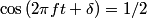$\cos \left(2 \pi f t + \delta \right) = 1/2$, so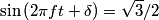$\sin \left(2 \pi f t + \delta \right) = \sqrt{3}/2$ or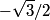$-\sqrt{3}/2$. So, the particle's speed is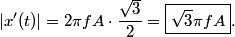\begin{align*}\left|x'(t) \right| = 2 \pi f A \cdot \frac{\sqrt{3}}{2} = \boxed{\sqrt{3} \pi f A}.\end{align*}Hence, answer (B) is correct.